# Homework 7¶

## Problem 1¶

In :
import numpy as np
import matplotlib.pyplot as plt

%matplotlib inline

In :
data = loadmat('data/mnist_data/images.mat')
X = data['images']


Here I implement Lloyd's algorithm...

In :
def lloyds(X, K):
# Create a dictionary to hold the clusters
cluster_nums = [j for j in range(K)]
clusters = dict((j, []) for j in cluster_nums)
means = dict((j, 0) for j in cluster_nums)
# Assign random initial clusters
for i in range(X.shape):
rand_cluster = np.random.randint(K)
clusters[rand_cluster].append(X[:, :, i])
# Begin algorithm
iteration = 0
while True:
for k in clusters:
mean = np.zeros((28, 28))
for point in clusters[k]:
mean += point
mean *= 1 / len(clusters[k])
means[k] = mean
new_clusts = dict((j, []) for j in cluster_nums)
for i in range(X.shape):
k = compute_MSE(X[:, :, i], means)
new_clusts[k].append(X[:, :, i])
truth_vals = [np.array_equal(clusters[k], new_clusts[k]) for k in clusters]
if not False in truth_vals:
return clusters, means
clusters = new_clusts
iteration += 1

def compute_MSE(X, means):
min_diff = (0, (X - means) @ (X - means))
for m in means:
diff = (m, (X - means[m]) @ (X - means[m]))
if np.linalg.norm(diff) < np.linalg.norm(min_diff):
min_diff = diff
return min_diff


Now I run Lloyd's for $k = 5, 10, 20$ and visualize the cluster centers. For $k = 10$ we more or less have a different digit for each cluster. Each digit clusters with itself. With $k = 5$ we only have half as many representatives, so we get clusters consisting of mainly two digits. For $k = 20$, we have roughly two clusters for each digit, so each image is placed in one of those two clusters based on whichever image it resembles most closely.

In :
clusters5, means5 = lloyds(X, 5)

In :
for center in means5:
print("Ceter for cluster %d" % center)
plt.figure()
plt.imshow(means5[center])

Ceter for cluster 0
Ceter for cluster 1
Ceter for cluster 2
Ceter for cluster 3
Ceter for cluster 4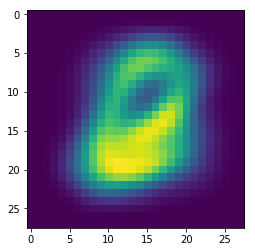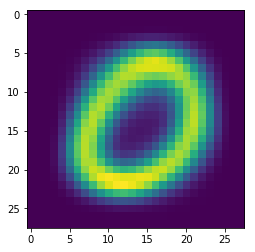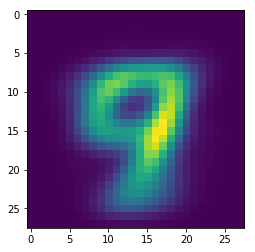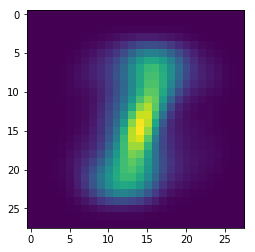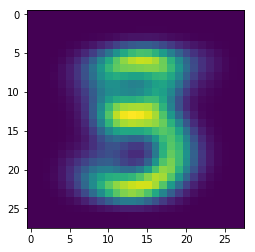In :
clusters10, means10 = lloyds(X, 10)

In :
for center in means10:
print("Center for cluster %d" % center)
plt.figure()
plt.imshow(means10[center])

Center for cluster 0
Center for cluster 1
Center for cluster 2
Center for cluster 3
Center for cluster 4
Center for cluster 5
Center for cluster 6
Center for cluster 7
Center for cluster 8
Center for cluster 9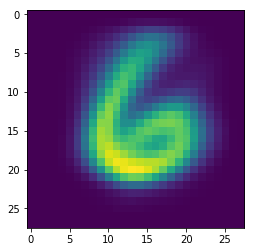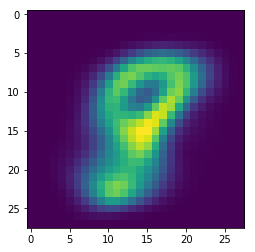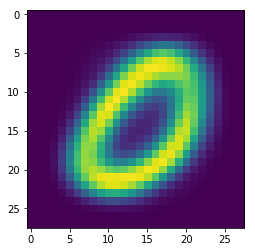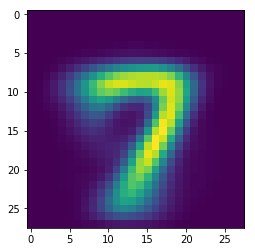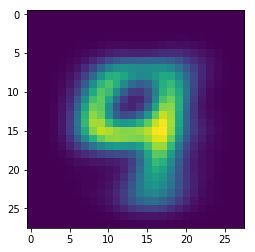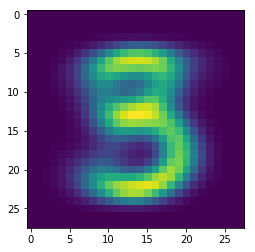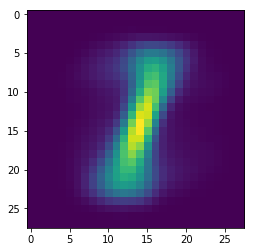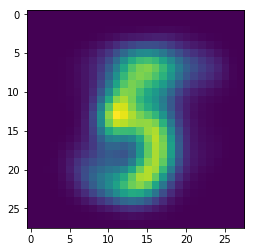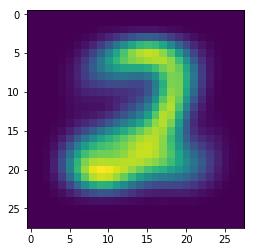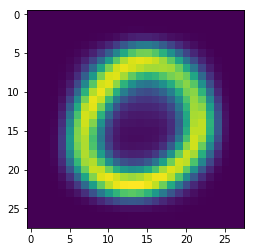In :
clusters20, means20 = lloyds(X, 20)

In :
for center in means20:
print("Center for cluster %d" % center)
plt.figure()
plt.imshow(means20[center])

Center for cluster 0
Center for cluster 1
Center for cluster 2
Center for cluster 3
Center for cluster 4
Center for cluster 5
Center for cluster 6
Center for cluster 7
Center for cluster 8
Center for cluster 9
Center for cluster 10
Center for cluster 11
Center for cluster 12
Center for cluster 13
Center for cluster 14
Center for cluster 15
Center for cluster 16
Center for cluster 17
Center for cluster 18
Center for cluster 19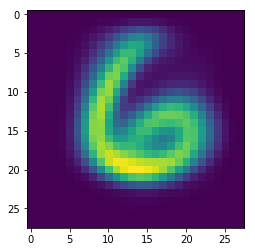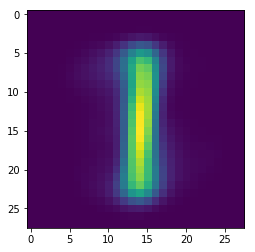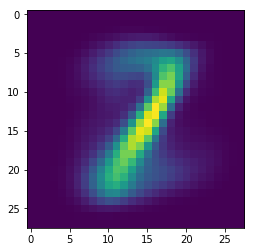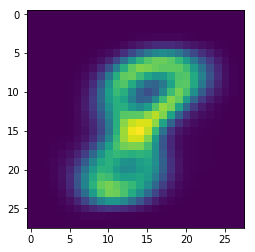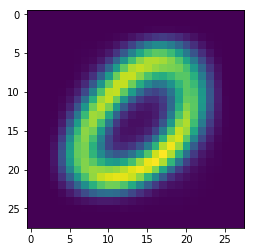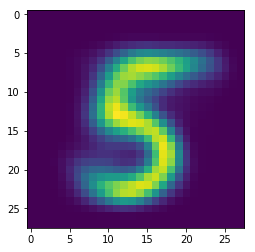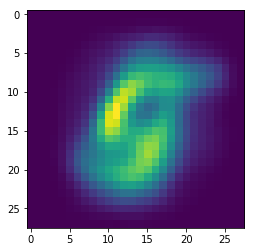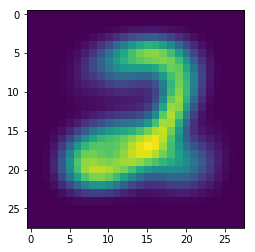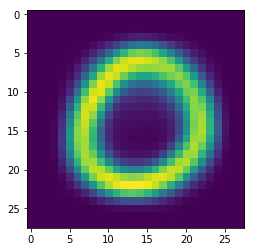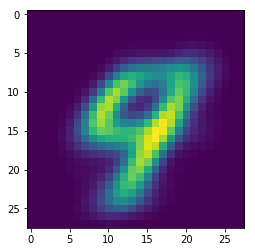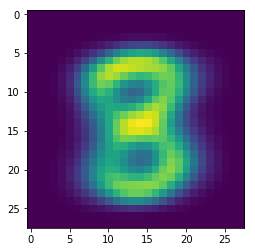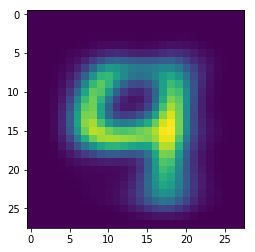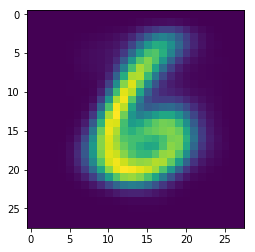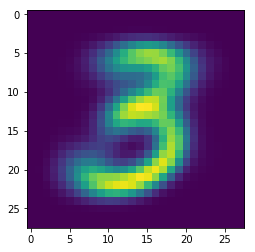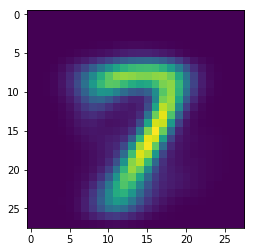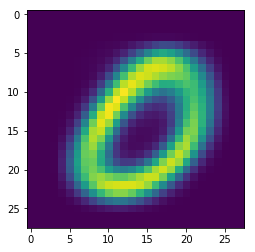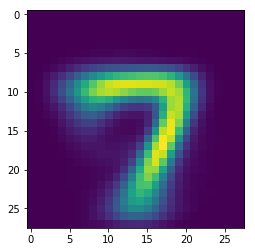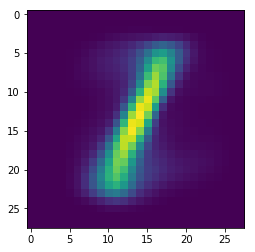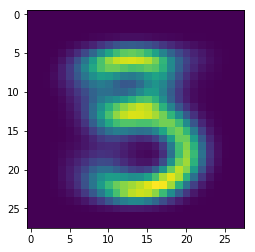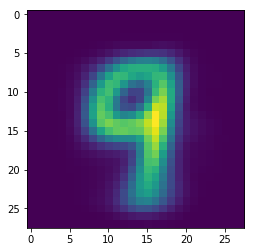## Problem 2¶

In :
from scipy.misc import imread
from sklearn.decomposition import TruncatedSVD

In :
face = imread('data/low-rank_data/face.jpg')


Here is the original face.

In :
plt.imshow(face);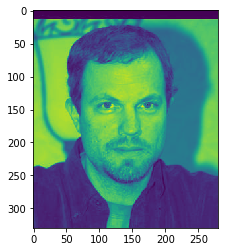Here is the rank-5 approximation.

In :
U, s, V = np.linalg.svd(face)
s[5:] = 0
S = np.zeros((330, 280))
S[:280, :280] = np.diag(s)
face5 = U @ S @ V
plt.imshow(face5);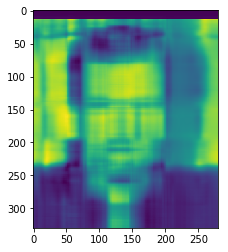Here is the rank-20 approximation.

In :
U, s, V = np.linalg.svd(face)
s[20:] = 0
S = np.zeros((330, 280))
S[:280, :280] = np.diag(s)
face20 = U @ S @ V
plt.imshow(face20);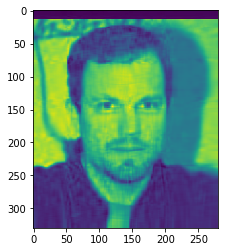And here is the rank-100 approximation.

In :
U, s, V = np.linalg.svd(face)
s[100:] = 0
S = np.zeros((330, 280))
S[:280, :280] = np.diag(s)
face100 = U @ S @ V
plt.imshow(face100);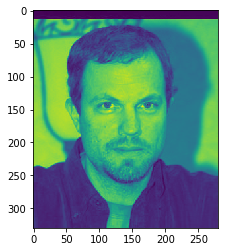Now I plot the mean squared error for the rank-i approximation, where i ranges from 1 to 100.

In :
def plotMSE(face, num_approx):
fig = plt.figure()
mses = []
xs = [i for i in range(num_approx)]
for i in range(num_approx):
mses.append(MSE(face, i))
ax.plot(xs, mses, 'b-')

def MSE(face, k):
U, s, V = np.linalg.svd(face)
s[k:] = 0
S = np.zeros((330, 280))
S[:280, :280] = np.diag(s)
facek = U @ S @ V
mse = np.linalg.norm(face - facek)
return mse

In :
plotMSE(face, 100)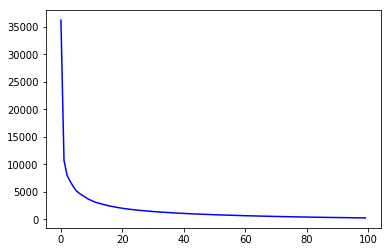Now, I compute the same rank approximations for the sky photo.

In :
img = imread('data/low-rank_data/sky.jpg')
plt.imshow(img);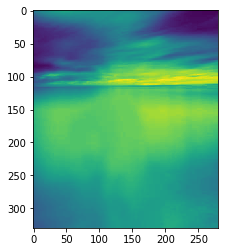In :
U, s, V = np.linalg.svd(img)
s[5:] = 0
S = np.zeros((330, 280))
S[:280, :280] = np.diag(s)
img5 = U @ S @ V
plt.imshow(img5);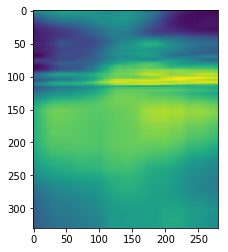In :
U, s, V = np.linalg.svd(img)
s[20:] = 0
S = np.zeros((330, 280))
S[:280, :280] = np.diag(s)
img20 = U @ S @ V
plt.imshow(img20);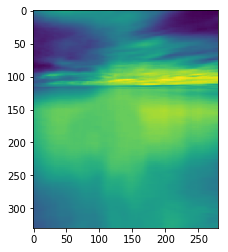In :
U, s, V = np.linalg.svd(img)
s[100:] = 0
S = np.zeros((330, 280))
S[:280, :280] = np.diag(s)
img100 = U @ S @ V
plt.imshow(img100);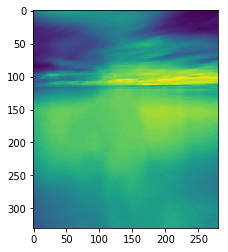I am unable to differentiate the rank-20 sky photo from the original, whereas with the face I need to get to the rank-100 approximation in order to not be able to differentiate it from the original. This is probably because in the sky photo, the colors run together and the level of detail is significantly less than for the face photo.

## Problem 3¶

In :
# Load in joke data

# Replace NaNs with 0
normalized_data = np.nan_to_num(joke_data['train'])


Here I compute the user and joke representations $U'$ and $V'$ in the following way. Let $U \Sigma V^T$ be the SVD of the data matrix. Then we have

$$U' = U \Sigma^{1/2}$$$$V' = \Sigma^{1/2} V^T$$
In :
# Compute SVD of R
U, s, V = np.linalg.svd(normalized_data, full_matrices=False)

# Fix up s into matrix form
S = np.zeros((100, 100))
S[:100, :100] = np.diag(s)

# Compute User Representation
users = U @ np.sqrt(S)
print(users.shape)

# Compute Joke Representation
jokes = np.sqrt(S) @ V
print(jokes.shape)

(24983, 100)
(100, 100)

In :
def plotMSE(joke, num_approx):
fig = plt.figure()
mses = []
xs = [i for i in range(num_approx)]
for i in range(num_approx):
mses.append(MSE(joke, i))
ax.plot(xs, mses, 'b-')

def MSE(joke, k):
U, s, V = np.linalg.svd(joke, full_matrices=False)
s[k:] = 0
S = np.zeros((100, 100))
S[:100, :100] = np.diag(s)
jokek = U @ S @ V
mse = np.linalg.norm(joke - jokek)
return mse


Now I plot the MSE as a function of $d$.

In :
plotMSE(normalized_data, 100)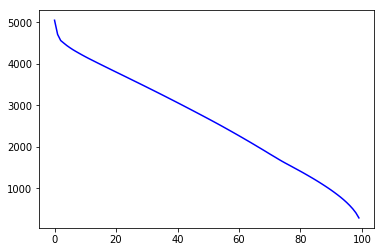In :
valid_set = np.loadtxt('data/joke_data/validation.txt', delimiter=',')
valid_set = valid_set.astype(int)
def validation_error(jokes, d):
U, s, V = np.linalg.svd(jokes, full_matrices=False)
s[d:] = 0
S = np.zeros((100, 100))
S[:100, :100] = np.diag(s)
R = U @ S @ V
R = np.where(R < 0, 0, 1)
valid_preds = valid_set[:, 2]
preds = R[valid_set[:, 0] - 1, valid_set[:, 1] - 1]
diff = preds - valid_preds
num_wrong = np.count_nonzero(diff)
return num_wrong / len(diff)

def plot_validation_error(jokes):
errors = []
xs = [i for i in range(100)]
for d in range(100):
err = validation_error(jokes, d)
errors.append(err)
fig = plt.figure()
ax.plot(